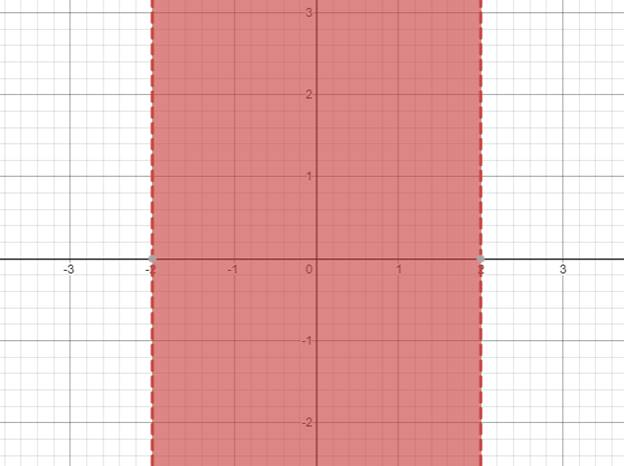# The solution of given nonlinear inequality equation and plot a graph for it.### Precalculus: Mathematics for Calcu...

6th Edition
Stewart + 5 others
Publisher: Cengage Learning
ISBN: 9780840068071### Precalculus: Mathematics for Calcu...

6th Edition
Stewart + 5 others
Publisher: Cengage Learning
ISBN: 9780840068071

#### Solutions

Chapter 1.7, Problem 47E
To determine

## The solution of given nonlinear inequality equation and plot a graph for it.

Expert Solution

2<x<2

### Explanation of Solution

Given:

Non-linear inequality equation

x2<4

Calculation,

Non-linear inequality equation,

x2<4x24>0(x2)(x+2)>0Now,x2>0x>2andx+2>0x<2so,2<x<2

Now, graph of x2<4Conclusion:

Hence, the values of x lies between the -2 and 2.

### Have a homework question?

Subscribe to bartleby learn! Ask subject matter experts 30 homework questions each month. Plus, you’ll have access to millions of step-by-step textbook answers!